# Constant Function

## Constant Function

Constant Function is defined as the real valued function $f : R \rightarrow R$ , y = f(x) = c for each $x \in R$ and c is a constant
So ,this function basically associate each real number to a constant value
It is a linear function where $f(x_1) =f(x_2)$ for all $x_1,x_2 \in R$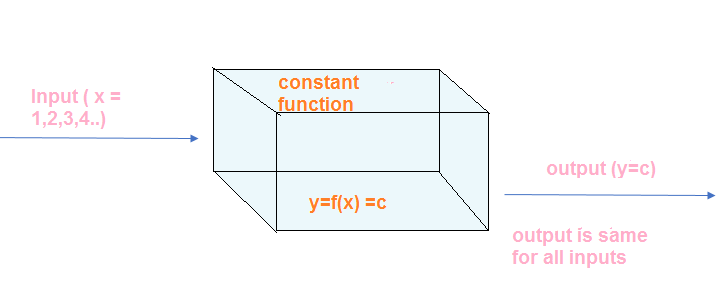## Domain and Range of the Constant Function

For $f : R \rightarrow R , y = f(x) = c$ for each $x \in R$

Domain = R
Range = {c}
The value of c can be any real number

## Graph of the Constant Function

We can draw the graph on the Cartesian plane with value of x on the x-axis and value of y=f(x) on the y-axis. We can plot the point and join the point to obtain the graph. Here in case of the constant function,the graph will be a straight line parallel to x-axis
It will be above x-axis if constant is positive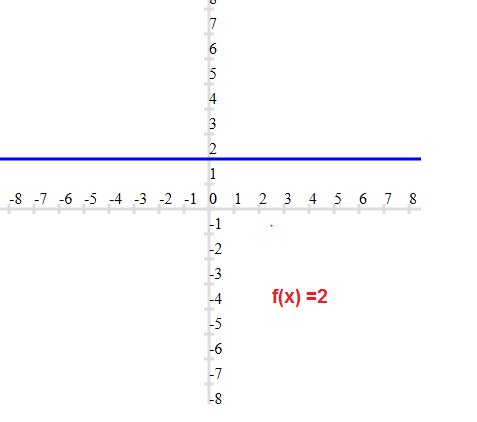It will be below x-axis if constant is negative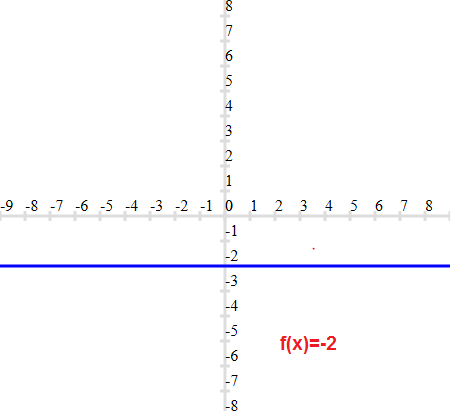It will be coincident with x-axis if constant is zero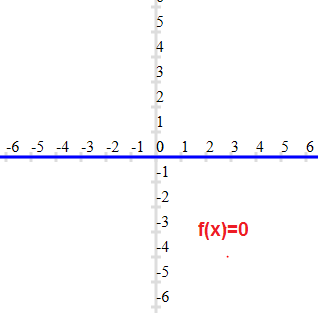It can concluded from above things above the ,the slope of constant function is 0

## Solved examples of constant Functions

1. which is below function is a constant function?
a. $y =x$
b. $y = 11$
c. $y=\pi$
d. $x + y=1$
e. $y =x^2$
Solution
For the function to be a constant function ,it should yield same value for each x
a. This is constant function as output is different for each input
b. This is constant function as output is same for each input. It is of the format y=c
c. This is constant function as output is same for each input. It is of the format y=c
d. This is constant function as output is different for each input
e. This is constant function as output is different for each input

2. which of the graph represent constant function?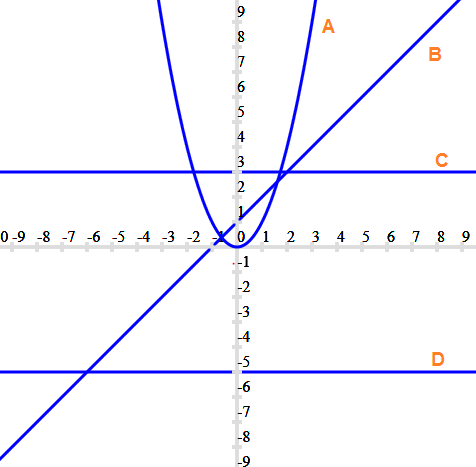Solution
The graph should be parallel to x-axis for the function to be constant function
So C and D are constant function

### Quiz Time

Question 1.
Find the value of the function $f(x) = \frac {x-5}{x-3}$ at x=0
A.3/5
B. 0
C.5/3
D. 1
Question 2.
If f (x) = px + q, where p and q are integers, f (-1) = - 5 and f (3) = 3, then p and q are equal to ?
A. p=2,q=-3
B. p-=2,q=3
C. p=1,q=3
D. p=-2,q=-3
Question 3.
if $f(x) =\frac {1}{2x+1}$ ,then
A. $f[f(x] =\frac {2x-1}{2x+3}$
B. $f[f(x] =\frac {2x-1}{2x-3}$
C. $f[f(x] =\frac {2x+1}{2x-3}$
D. $f[f(x] =\frac {2x+1}{2x+3}$
Question 4.
Let $f(x) =c$,find the value of $f(2) -f(1)$
A. 4
B. 2
C. 0
D. 1
Question 5.
The slope of the constant function is
A. 0
B. 45
C. 90
D. 180
Question 6.
Find the Range and domain of the function $f(x) =-\frac {1}{2}$
A. Domain = R, Range =R
B. Domain = R - {0}, Range = R
C. Domain = R , Range = {-1/2}
D. Domain = R - {1}, Range = R